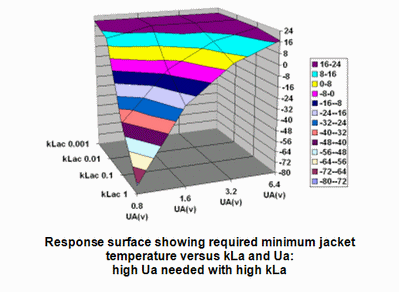## Friday, February 8, 2008

### Design Space for a Synthesis Reaction, Part 2

Results below are taken for the purposes of illustration from a predictive mechanistic model of the hydrogenation in the preceding post. The model represents the user's process scheme (including the proposed reaction scheme) as rate equations, then integrates the component balances for each phase and the energy balance over the cycle time of the operation.

The equations are from classical chemical engineering and 'differential', i.e. the model calculates the rate of change with respect to time of each variable from a starting point, the initial value. That initial value comes from the experimental conditions and is a familiar 'factor' setting such as temperature, concentration, pressure. The equations include scale-independent rate constants for reactions (k) and scale-dependent rate constants for mass transfer (kLa) and heat transfer (Ua), each of which has been fitted to lab or plant data, or estimated using a predictive technique (such as a chemical engineering equipment correlation).

When fitting parameters, the model must fit not just the end-points, but also the shapes of the measured profiles; this strict criterion means that the iterative process of fitting the parameters encourages development of genuine process understanding and produces a model that can within reason be extrapolated as well as interpolated to anticipate how the reaction will respond on scale-up or when other changes (e.g. to the recipe and conditions) have to be made.

Results for a short series of 'screening' batches in which the recipes, conditions and scale-dependent rate constants have been varied over practical ranges are shown below. Compared to experimentation, many more scenarios can be considered with the model ("electrons are cheap"); here, there is typical sensitivity to temperature, pressure, substrate concentration, catalyst loading and kLa. Visualizing effects in several dimensions can be a challenge; here the ribbon plot provides a quick overview.An important point is that these results are now representative of operation at any scale, large or small, as scale-dependent rate constants have been included to capture equipment effects.

A conventional (statistical) design of experiments to map out the design space might consider ranges of temperature, pressure, substrate level, catalyst loading, agitation and reaction time as factors in a design, with a view to defining the 'corners' defined by the acceptable ranges for these settings. As pointed out in the preceding post, this non-mechanistic approach to the scale-dependent factors does not recognize that for example the required mass transfer rate constant depends on each of the first four factors and that the required heat transfer rate constant depends on all five of the other factors and the available minimum jacket temperature, which is also scale-dependent. Using the mechanistic model, each of these parameters can be varied systematically over their actual likely ranges at any scale to see the impact on CQA. We are not confined to any type of limited factorial design, in which we have to change several factors at a time to minimize the experimental programme; we can cover the whole space of interest quickly and comprehensively.

Sample response surface plots generated by the model are shown below for i) impurity level versus kLa and catalyst loading ii) reaction time versus the same factors; iii) a composite plot of the deviation of both impurity and reaction time from target values (here, 10% and 100 minutes, respectively), versus the same factors and iv) the required minimum jacket temperature versus kLa and Ua.The above all sounds good, but there is better to come. In defining the Design Space, why restrict ourselves at all to any specific ranges of any of the CPPs, when we know the acceptable ranges depend on each other and that hardwired definitions will reduce our operational flexibility? If we possess a verified mechanistic model that links CQA to each of the CPPs, the design space could be any combination of CPPs that produce an acceptable CQA. In other words, we can operate with potentially extreme or very limited values of certain CPPs as long as we adjust other CPPs to compensate; the model tells us whether compensation is possible and if so, how to achieve it.

Further posts will consider how broad that design space might be and what constitutes model verification for the purposes of defining the design space in this flexible way. All results presented in this post were generated using DynoChem software.